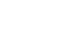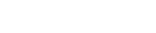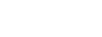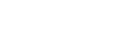## Mathematics Paper 1 Questions and Answers - Maranda Mock Examinations 2022

Instructions to Candidates

1. This paper consists of two sections; Section I and Section II.
2. Answer all the questions in Section I and any five questions from Section II
3. Show all the steps in your calculations, giving your answers at each stage in the spaces provided below each question
4. Marks may be given for correct working even if the answer is wrong.
5. Non-programmable silent electronic calculators and KNEC Mathematical tables may be used, except where stated otherwise.

### QUESTIONS

Section I (50 Marks)
Answer ALL questions in the section in the space provided:

1. Evaluate without using a calculator 0.01% of. (3 marks)
2. The sum of the ages of three brothers; Mike, Charles and Abdul is 65 years. Mike is twice as old as Abdul and one and half times as old as Charles. Determine their ages. (3 marks)
3. A supermarket has 18 apples, 30 oranges and 54 apples. The fruits are to be arranged in rows such that every row contains same number of fruits of one type only. Determine the least number of rows required for this arrangement to happen. (3 marks)
4. Find the area of the rectangle below. (3 marks)5. Without using a Calculator or Mathematical Table evaluate the value of p: (3 marks)
4/P2 =2212- 2202
6. Calculate the value of x given thatand |AB|= 5 units. (3 marks)
7. Solve for k in the equation. (2 marks)
8. Solve for x in the equation(3 marks)
9. In the figure below ABF is a uniform cross section of a solid. AB, BC and BF are some of the visible edges of the solid. Complete the sketch showing the hidden edges with broken lines. (3 marks)10. Mr. Charles who deals in electronics sells a radio to a customer at Kshs. 1,440 after giving him a discount of 10% but finds that he still makes a 20% profit. Find the profit Mr. Charles would make if he does not give a discount. (3marks)
11. The figure below shows a set of two right angled triangles ABD and BCD conjoined by side BD. Sides AB, BC and AD are 6cm, 5cm and 10cm in lengths respectively.Calculate the size of angle CDB=t. (4 marks)
12. Given that log⁡3= 0.4771 and log5 = 0.6990, find without using Mathematical table or calculator log 0.045. (3 marks)
13. Two towns A and B are 810km apart. A car left A at 8:00am moving towards B at an average speed of xkm/h. A bus left town B at 9:30am moving towards A at an average speed of 84km/h. Determine the average speed of the car, xkm/h, if the two vehicles met at 11.00am. (4 marks)
14. In the figure given below, O is the centre of circle. If ∠BCA= 80º and ∠CB0= 10º.Determine the size of ∠CAB. (3 marks)
15. Using mid-ordinate rule with six strips estimate the area between the curve y=3x2+4x+10, x-axis and the lines x=-2 and x=4. (4 marks)
16. Construct A'B'C'D' the image of quadrilateral ABCD below. Given that it undergoes under transformation enlargement with scale factor -2 and centre O. (3 marks)Section II (50 Marks)
Answer ONLY FIVE questions in the section in the space provided:
17. The equation of line L1 is 2x+3y+ 6= 0.
1. Find the gradient of line L1 (1 mark)
2. The line l2 is perpendicular to l1 and passes through the point P with coordinates (7, 2), find the equation of l2 , giving the answer in the form y= mx+ c where m and c are constants. (3 marks)
3. The line l1 and l2 intersect at point Q. Find the coordinates of Q. (3 marks)
4. The line l3 is parallel to l1 and passes through point P. Find the equation of l3 giving the answer in the form ax+ by = c , where a , b and c are constants. (2 marks)
5. Determine the x and y-intercepts of the line l3. (1 mark)
18. The Form Two Parents of Ushindi High School decided to buy laboratory equipment worth Kshs.900,000 by each student contributing equal amount of money. Before they made the contribution 50 students got transferred to other institutions and this made the remaining students to pay Kshs.600 more.
1. Taking to represent the original number of students, write down an expression for:
1. The original contribution per student. (1 mark)
2. The new contribution per student. (1 mark)
2. Find the original number of students who were to contribute. (4 marks)
3. Calculate the percentage change in the students’ contributions. (2 marks)
4. If the ratio of boys to girls that remained in Form 2 was 13:12 calculate the amount contributed by the boys alone. (2 marks)
19. Two circles of radii 3.5 and 4.2 cm with centres O1 and O2 respectively intersect at points A and B as shown in the figure below. The distance between the two centres is 6 cm and the distance from O1 to the common chord AB along O1O2 is x cm.Calculate:
1. The distance x cm to 3 decimal places. (3 marks)
2. The size of ∠AO1B and ∠AO2 B (to the nearest degree). (3 marks)
3. The area of quadrilateral O1AO2B, correct to 2 decimal place (2 marks)
4. The shaded area correct to two significant figures. (Take. π=3.142). (2 marks)
20. Three towns A, B and C are such that B is 120km on a bearing of 060º from A. C lies directly East of A on a bearing 165º from B. Using a scale of 1cm to represent 20km, a pair of compass and a ruler only, determine:
1. the relative positions of A, B and C. (3 marks)
2. drop a perpendicular from B to meet AC at M hence measure BM. (2 marks)
3. construct a circle passing through the vertices A, B and C then calculate the area covered in the circle. (2 marks)
4. Determine the amount of money used in ploughing the land inside the circle but outside triangle ABC at a rate of Kshs. 5000 per hectare. (3 marks)
21. A solid is partly a cone and partly an hemisphere of radius 7cm. The slanting length of the cone is 25cm. Determine;
1. the height of the solid. (2 marks)
2. the volume of the solid (3 marks)
3. the surface area of the solid. (3 marks)
4. the mass of the solid if its density is 12.5g/cm3. (2 marks)
22. In the figure below OB = b; OC = 3OB and OA = a
1. Given that OD = 1/3OA and AN = 1/2AC, CD and AB and and meet at M. Determine in terms of a and b.
1. AB
2. CD
2. Given that CM = kCD and AM = hAB. Determine the values of the scalars k and h. (5 marks)
3. Show that O, M and N are collinear. (3 marks)
23. The displacement of a particle is given as s=t3- 6t2+9t+50 metres.
Determine;
1. The displacement of the particle when t = 2 seconds. (2 marks)
2. the velocity of the particle when t = 4 (2 marks)
3. the acceleration of the particle when t = 5. (2 marks)
4. the time when the particle is at rest. (2 marks)
5. the displacement during the 3rd second. (2 marks)
24. The height of a number of students was recorded in the table below. Each measurement is given to the nearest cm.
 Height(cm) Mid-point x Frequency 138-142 140 3 420 143-147 8 148-152 12 153-157 20 158-162 160 30 4800 163-167 14 168-173 7 173-177 4 178-182 180 2 360 ∑f = ∑fx =
1. Complete the table. ` (3 marks)
2. use the completed table to calculate the mean height of the students. (2 marks)
3. Draw a histogram to represent the information. (2 marks)
4. Use the histogram above to estimate the median height. (3 marks)

### MARKING SCHEME

1.2. M: C : A = 2x ; 3x ; 1.5x
x = 65/13
x = 5
mike's age = 30 years
charles age = 20 years
abdul's age = 15 years
3. 18 = 2 x 32
30 = 2 x 3 x 5
54 = 2 x 33
gcd = 2 x 3
= 6
no of rows = 18/6 + 30/6 + 54/6
= 17
4. 2x + 6 = x + 8
x = 2
L = 21 and W = 10
A = 21 x 10
= 210
5. 4/p2 = (221 - 220)(221 + 220)
1/p2 = 441/4
1/p = ±21/2
p = ±2/21
6. AB = AO + OB
ans = x = 1 or 9
7. 2k + 10 + 3k - 20 = 90º
k = 20º
8. √-2x - 6 = 3 + x
-2x - 6 = x2 + 6x + 9
x2 + 8x + 15 = 0x = -5 or -3
9.10. M.P. = 1440 x 100
90
= 1600
S.P = 1440 x 100
120
= 1200
profit = 1600 - 1200
= 400
11. BD = √102 + 102
= 11.662
sin CDB =       5
11.662
= 28.209º
12. log 0.045 = log(32 x 5 x 10-3)
= 2log3 + log5 + log10-3
= 2 x 0.4771 + 0.6990 + 3
= 2.6532
13. The distance covered by the car by 9:30 am = 1.5xkm
Remaining distance = 810 - 1.5x
810 - 1.5x = 1.5
x + 84
810 - 1.5x = 1.5x + 126
x = 228
14. <OAC = <OCA = 70º and <OAB = 10º
<CAB = 70º + 10º
= 80º
15.
 x -1.5 -0.5 0.5 1.5 2.5 3.5 y 10.75 8.75 12.75 22.75 38.75 60.75
A = 1(10.75 + 8.75 + 12.75 + 22.75 + 38.75 + 60.75)
= 154.5
16.17.
1. 3y = -2x - 6
y = -2/3x - 2
m1 = -2/3
2. -2/3 x m2 = -1
m2 = 1.5
y - 2 = 1.5
x - 7
y = 1.5x - 8.5
3. at Q - 2/3x - 2 = 1.5x - 8.5
x = 3
y = -2/3 x 3 - 2
= - 4
hence the co-ordinates of is Q(3, -4)
4. m3 = -2/3
y - 2 = -2
x - 7     3
2x + 3y = 20
5. y intercept is 62/3 and x intercept is 10
18.
1.
1. 9000/x
2. 90000
x - 50
2. 90000 - 90000 = 600
x - 50        x
600x2 - 30000x - 45000000 = 0
x = 50 ± √2500 + 300000
2
= 50 ± 550
2
= 300 or - 250
∴x = 300
3. original contribution = 900000/300
= 3000
%change = 600 x 100%
3000
= 20%
4. remaining students = 250
boys contributions = 130 x 3600
= 468 000
19.
20.
1. scale diagram2. 3.0 ± 0.1 cm
60 ± 2 km
3. radius 3.2 ± 0.1 cm
A = 22/7 x 642
= 12873.142857142 km2
4. Area = 12873.142857142 - 1/2 x 120 x 60
= 9273.142857142
money spen = 9273.142857142 x 106 x 5000
104
= kshs. 4636571429
21.
1. h = 7 + √252 - 72
= 31 cm
2. v = 1/3 x 22/7 x 72 x 24 + 2/3 x 22/7 x 73
= 19502/3
3. S.A = 2 x 22/7 x 72 + 22/7 x 7 x 2
= 858
4. m = 12.5 x 19502/3
= 243831/3g
22.
1.
1. AB = -a + b
2. CD = 1/3a - 3b
2. k = 3/4 and h = 3/4
3. OM = MN hence OM is parallel MN
Given OM is parallel MN and they share a common point M then points O, M and N are collinear
23.
1. S(2) = 23 - 6 x 22 + 9 x 2 + 50
= 52
2. v = 3t2 - 12t + 9
v = 3 x 42 - 12 x 4 + 9
= 9
3. a = 6t - 12
when t = 5a = 6 x 5 - 12
a = 18
4. at rest v = 0
v = 3t2 - 12t + 9 = 0
t = 1s or 3s
5. S(3rd) = 33 - 6 x 32 + 9 x 3 + 50) - (23 - 6 x 22 + 9 x 2 + 50)
= -2m
24.
1.
 height (cm) mid-point (x) frequency (f) fx area cummulative area 138 - 142 140 3 420 15 15 143 - 147 145 8 1160 40 55 148 - 152 150 12 1800 60 115 153 - 157 155 20 3100 100 215 158 - 162 160 30 4800 150 365 163 - 167 165 14 2310 70 435 168 - 172 170 7 1190 35 470 173 - 177 175 4 700 20 490 178 - 182 180 2 360 10 500 Σf = 100 Σfx = 15840
2.
x = 15840/100
=158.4 cm
3.4.
1/2 cummulative area = 250
hence the median class is 138 - 162
30x = 250 - 215
x = 11/6
median = 157.5 + 11/6
= 158 2/3

• ✔ To read offline at any time.
• ✔ To Print at your convenience
• ✔ Share Easily with Friends / Students